# 978-0134292380 Chapter 9

Document Type
Test Prep
Book Title
Fundamentals of Hydraulic Engineering Systems 5th Edition
Authors
A. Osman H. Akan, Ned H. C. Hwang, Robert J. Houghtalen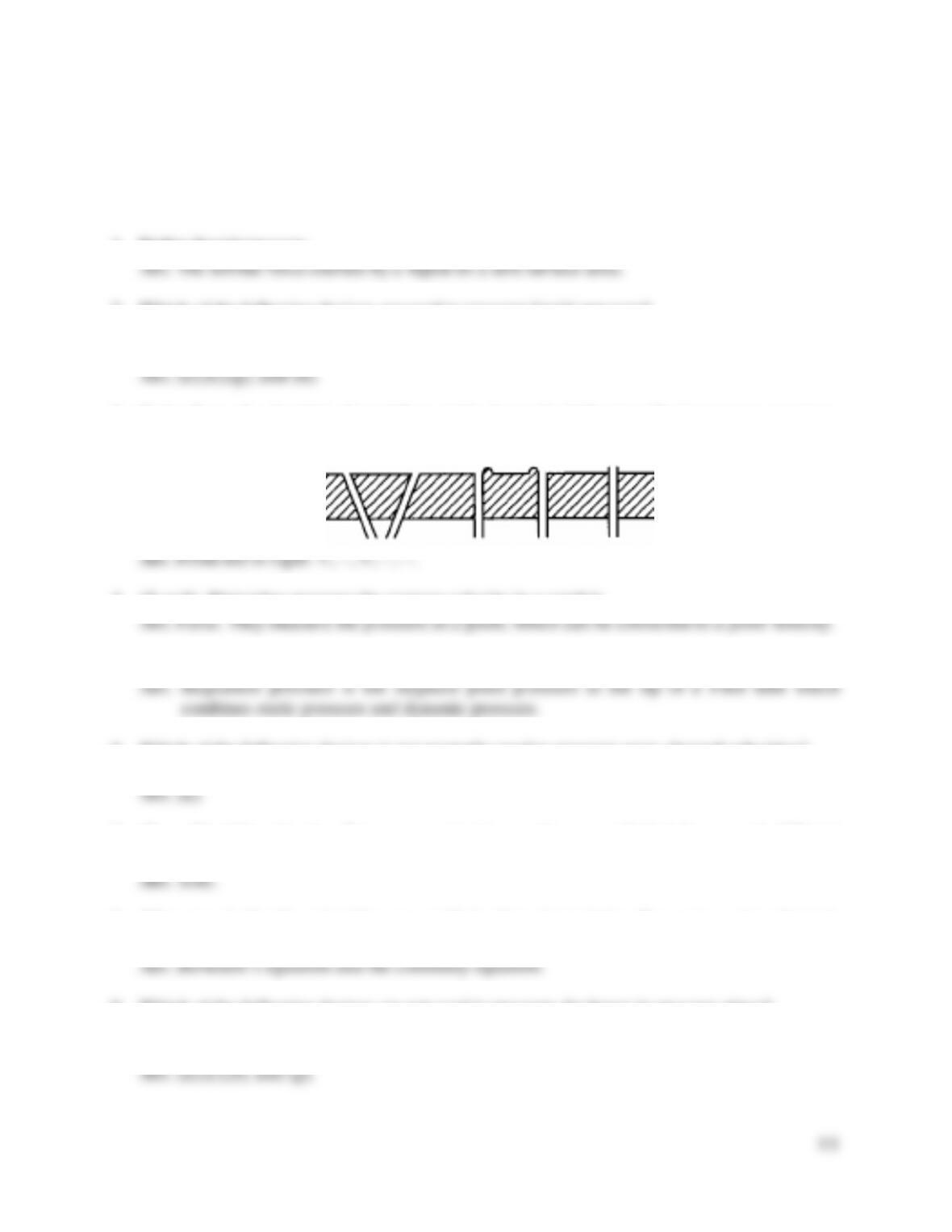88
TEST QUESTIONS & PROBLEMS - CHAPTER #9
1. Define liquid pressure.
2. Which of the following devices are used to measure liquid pressure?
a) Pitot tube b) Venturi meter c) propeller-type meter d) orifice meter
e) manometer f) Parshall flume g) Bourdon tube gauge h) transducer
3. If the flow of a liquid is from left to right above the following (five) pressure openings,
identify whether the pressure will register too high (+) or too low (-).
4. (T or F) Pitot tubes measure the average velocity in a conduit.
5. Define stagnation pressure.
6. Which of the following devices is not normally used to measure open-channel velocities?
a) Pitot tube b) cup-type meter c) propeller-type meter d) acoustic velocity meter
7. (T or F) Although pipe flow measurements can be accomplished by several different
methods, the simplest and most reliable measurement is the volumetric (or weight) method.
8. What two hydraulic principles are applied when determining flow rates using Venturi,
nozzle, and orifice meters?
9. Which of the following devices are not used to measure discharge in pressure pipes?
a) Pitot tube b) Venturi meter c) propeller-type meter d) orifice meter
e) bend meter f) Parshall flume g) Bourdon tube gauge h) nozzle meter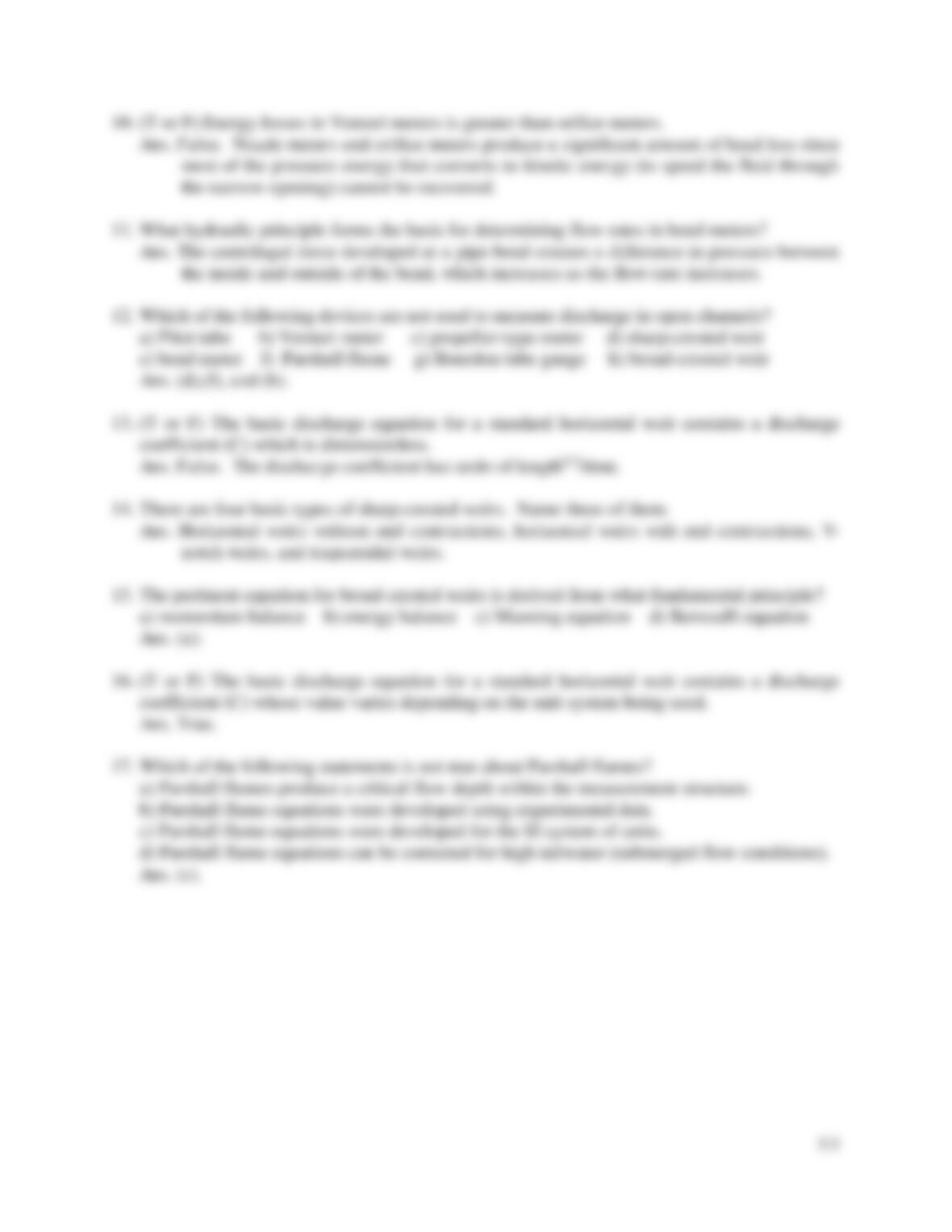10. (T or F) Energy losses in Venturi meters is greater than orifice meters.
11. What hydraulic principle forms the basis for determining flow rates in bend meters?
12. Which of the following devices are not used to measure discharge in open channels?
a) Pitot tube b) Venturi meter c) propeller-type meter d) sharp-crested weir
e) bend meter f) Parshall flume g) Bourdon tube gauge h) broad-crested weir
13. (T or F) The basic discharge equation for a standard horizontal weir contains a discharge
coefficient (C) which is dimensionless.
14. There are four basic types of sharp-crested weirs. Name three of them.
15. The pertinent equation for broad-crested weirs is derived from what fundamental principle?
a) momentum balance b) energy balance c) Manning equation d) Bernoulli equation
16. (T or F) The basic discharge equation for a standard horizontal weir contains a discharge
coefficient (C) whose value varies depending on the unit system being used.
17. Which of the following statements is not true about Parshall flumes?
a) Parshall flumes produce a critical flow depth within the measurement structure.
b) Parshall flume equations were developed using experimental data.
c) Parshall flume equations were developed for the SI system of units.
d) Parshall flume equations can be corrected for high tailwater (submerged flow conditions).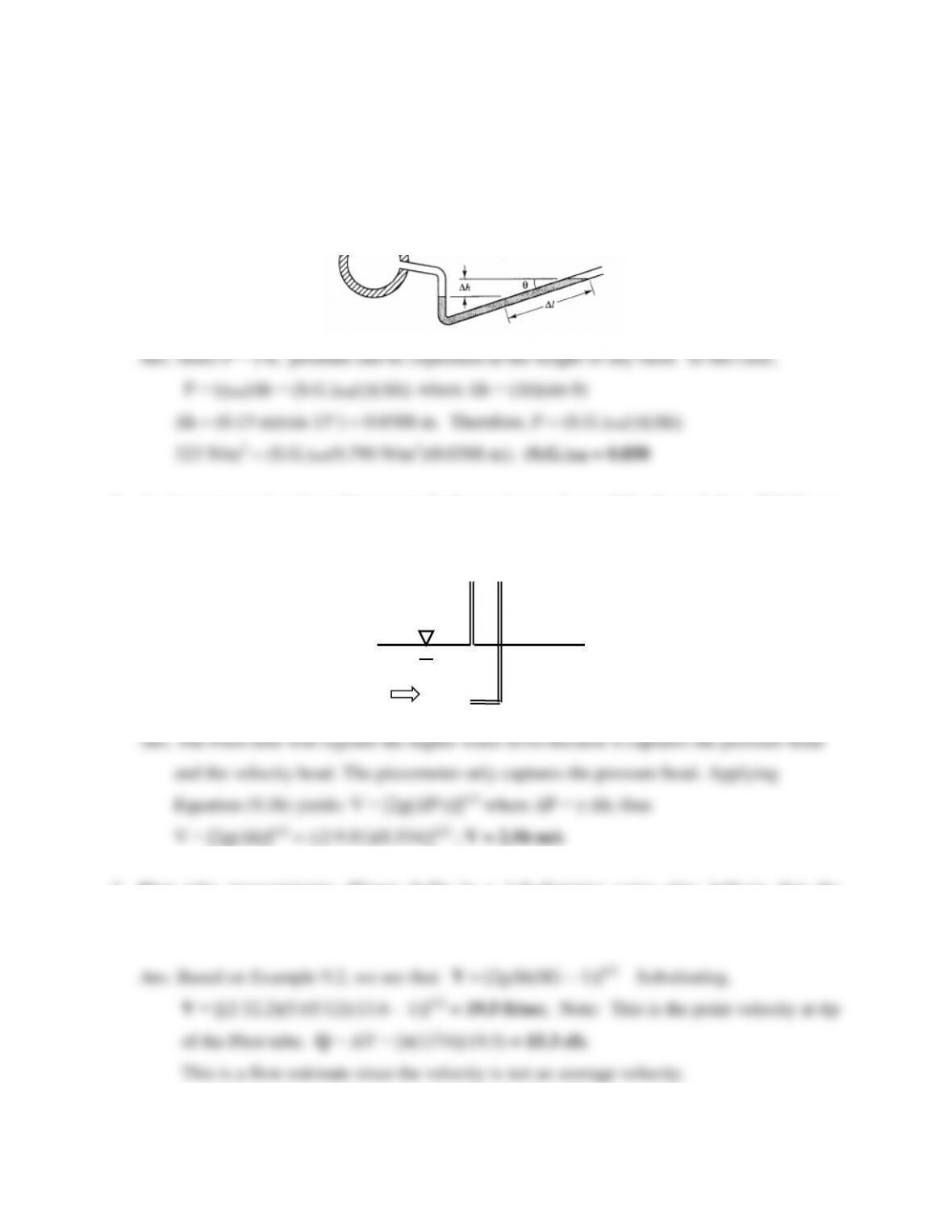90
Problems
1. An inclined oil manometer (shown below) is used to measure pressure in a wind tunnel.
Determine the specific gravity of the oil (shaded color) if a pressure change of 323 Pascals
occurs causing a 15-cm reading change on the 15° incline.
2. A piezometer and a pitot tube are attached to a pipe as shown if the figure below. Which one
will record the higher water level? Explain. Also determine the flow velocity in the pipe if
the difference in the water levels between the piezometer and the pitot tube is 34.4 cm.
3. Pitot tube measurements (Figure 9.4b) in a 1-ft-diameter water pipe indicate that the
stagnation pressure and the static pressure differ by a mercury column height of 5.65 inches.
Estimate the discharge coming through the pipe. Why is it just an estimate of discharge?
Pitot tube
Piezometer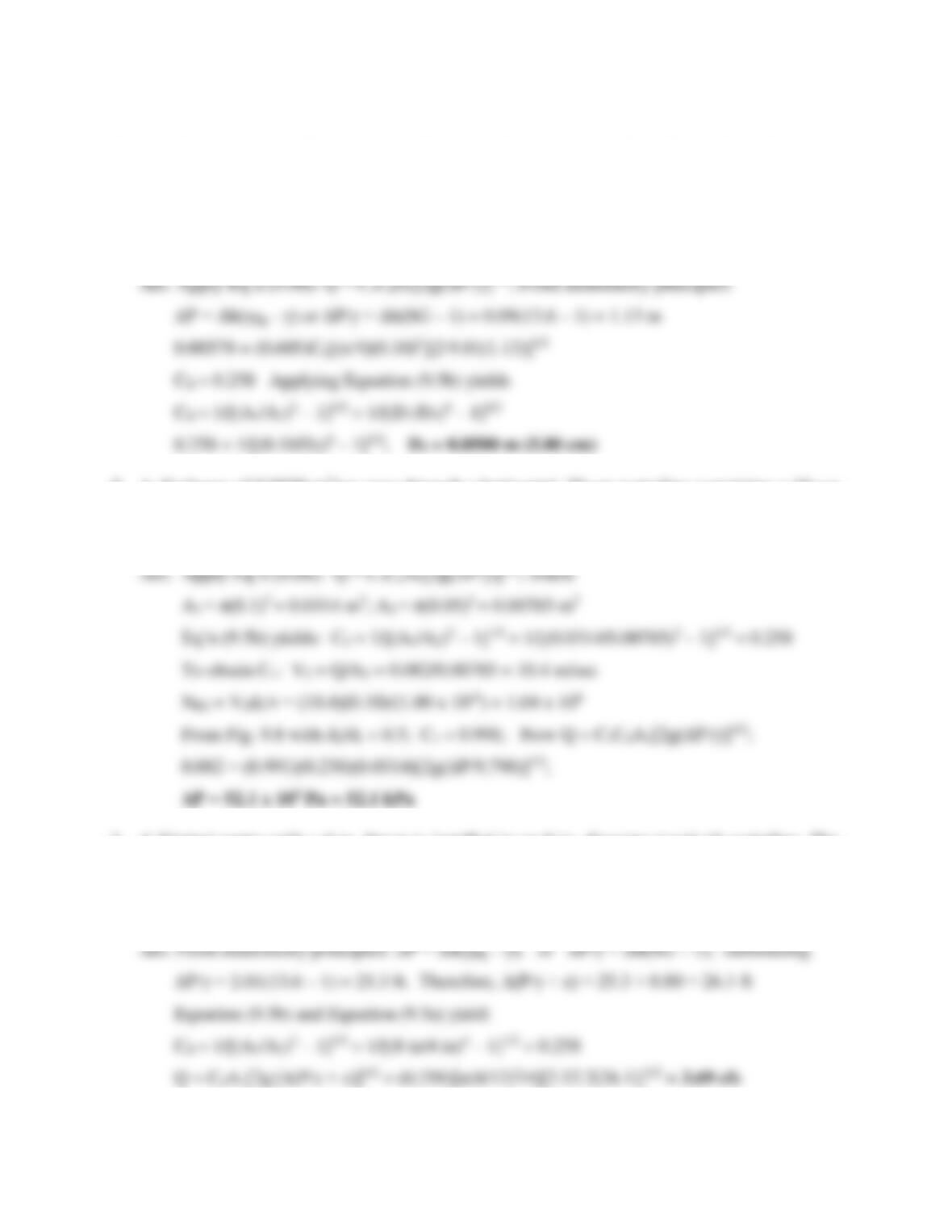91
4. A maintenance crew discovers an orifice meter in an old water line. The engineer in charge
would like to know the size of the orifice plate inside without dismantling the delicate meter.
A specification plate on the side of the meter reads, Cv = 0.605. For a flow rate of 0.00578
m3/sec, the attached mercury-water manometer registers a pressure difference of 9.0 cm. If
the horizontal pipeline has an inside diameter of 10 cm, what is the size of the orifice plate?
5. A discharge of 0.0820 m3/sec pass through a horizontal, 20-cm waterline containing a 10-cm
ASME flow nozzle. Determine the pressure difference that will register across the flow meter
in Pascals.
6. A Venturi meter with a 4-in. throat is installed in an 8-in. diameter (vertical) waterline. The
pressure difference reading between the throat and entry section in a mercury-water
manometer is 2.01 feet. Determine the flow rate if the flow is moving downwards and the
distance between the pressure taps is 0.80 feet. SG (mercury) = 13.6.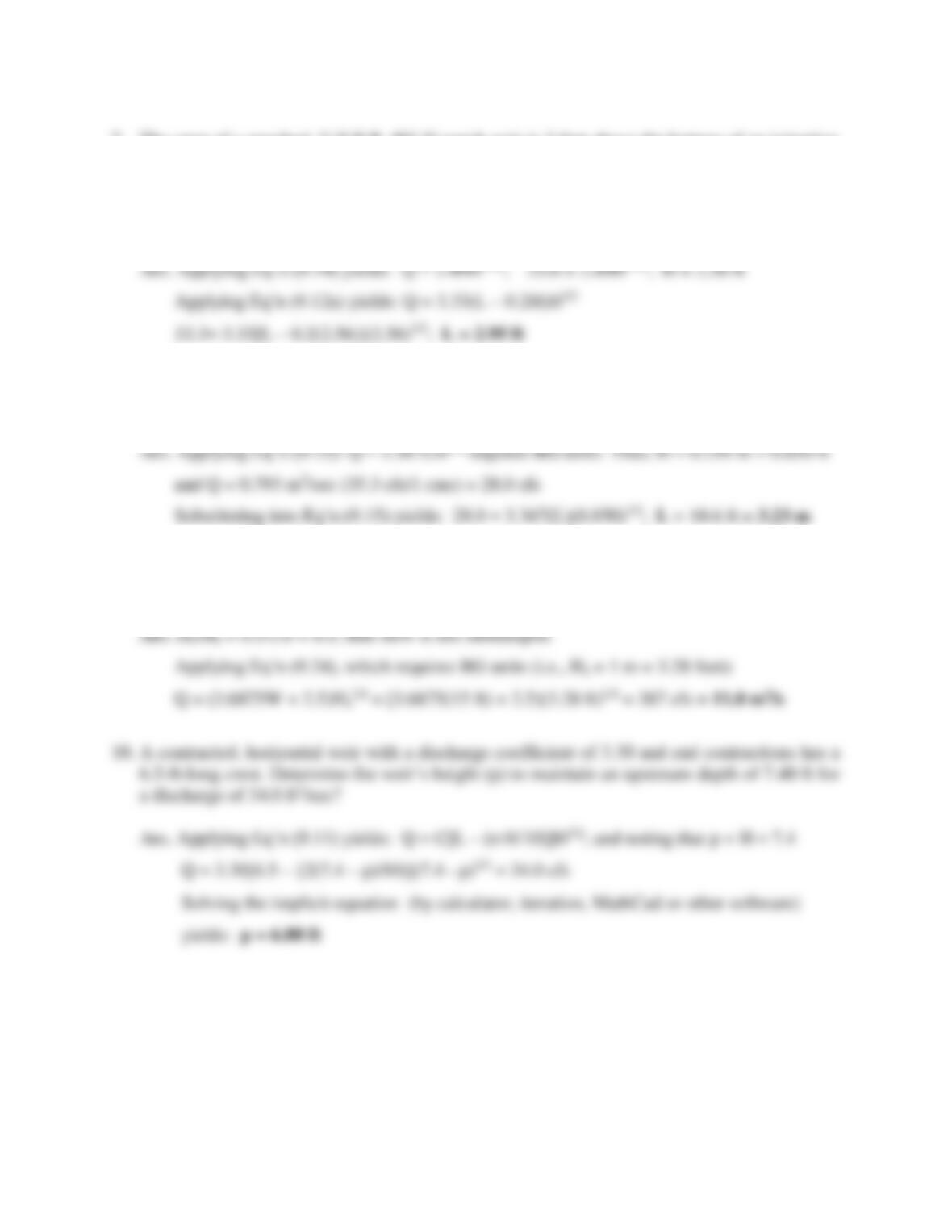92
7. The crest of a standard, U.S.B.R. 90°-V-notch weir is 3 feet above the bottom of an irrigation
channel. It measures 25.6 cfs of flow when the upstream water depth is at the top of the
channel bank. An increased flow rate (33.3 cfs) is needed without flooding the upstream
channel. Determine the required length of a replacement weir (USBR standard contracted
horizontal weir with the same crest height) to accommodate the new flow demand.
8. What is the length of the Cipolletti weir (U.S.B.R. standard trapezoidal weir) required to
accommodate a flow up to 0.793 m3/sec if the maximum head is limited to 0.259 m?
9. Determine the discharge in cubic meters per second measured by a 15-ft Parshall flume if the
gage reading Ha is 1 m and the gage reading Hb is 0.5 m.

## Trusted by Thousands ofStudents

Here are what students say about us.